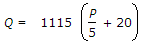# Civil Engineering - Water Supply Engineering - Discussion

### Discussion :: Water Supply Engineering - Section 1 (Q.No.19)

19.

Most satisfactory formula for an estimate of fire demand Q for a city of population P in thousands for Indian conditions, is

 [A].[B]. Q = 1640 P (1 - 0.01 P) [C]. Q = 3180 P [D]. none of these.

Explanation:

No answer description available for this question.

 Amar Sathe said: (Jul 27, 2015) Q = 3182 under root p.

 Nani said: (Sep 18, 2015) According to Indian standard formula it is B.

 P S Thanveer said: (Feb 13, 2016) It's known as Kuchling's Formula & its used when population exceeds 50, 000.

 Uchit said: (Jun 24, 2017) I think b would be more appropriate because Indian standard code recommend it to be used in Indian condition.

 Ashish Tiwari said: (Jul 3, 2017) Actually formula a and b are wrong It's 1136(p/5 +10). 4637under root p(1-0.01under root p). That's why answer is C? If above formulas were right then and must be b because it is suggested by Indian standards

 Dvs said: (Aug 24, 2017) Yes, @Ashish. 5 correct.

 Mahidip said: (Sep 23, 2017) Kuchling's formulae is appropriate and most satisfactorily to measure fire demands.

 Akshay said: (Jun 14, 2018) 3182 √p -> Kuchling's Formula.

 Amar said: (Sep 3, 2018) Actual formula 3182√ P. None of the above answer is correct.

 Prabhat said: (Sep 22, 2018) Yes, Option D (none of the above answers is correct). Due to Kuching's Formula: Q=3182 X √P.

 Pari said: (Jun 6, 2019) Kuchling's formula. Q=3182 * √ P, where P is in thousands and Q in litres/min.

 Sipashree Behera said: (Jun 9, 2019) Yes, Q=3182*√P.

 K.Nandini said: (Sep 20, 2019) It should be Q=3182 √p.

 Suraj said: (Sep 4, 2020) Q = 3182 √P.